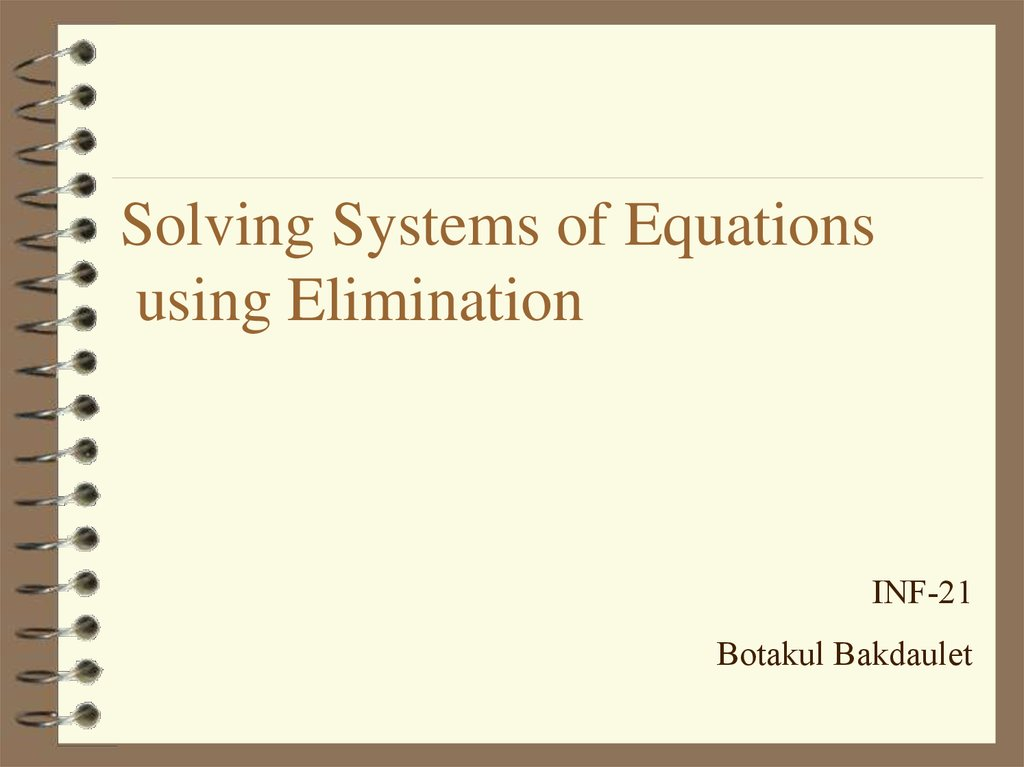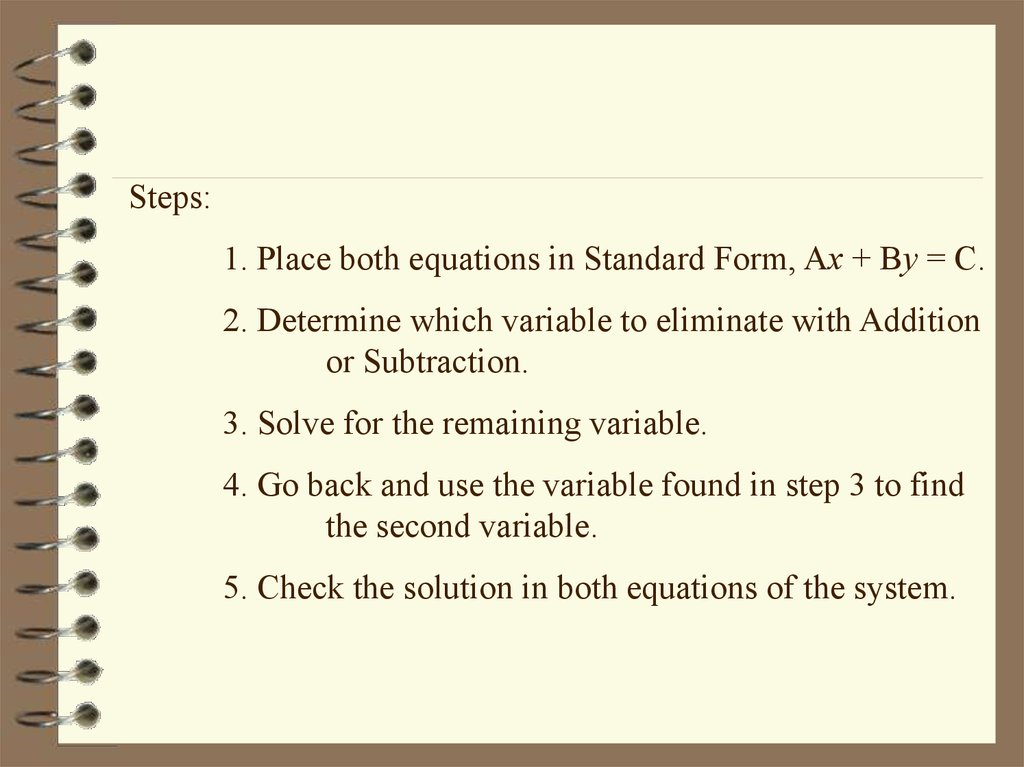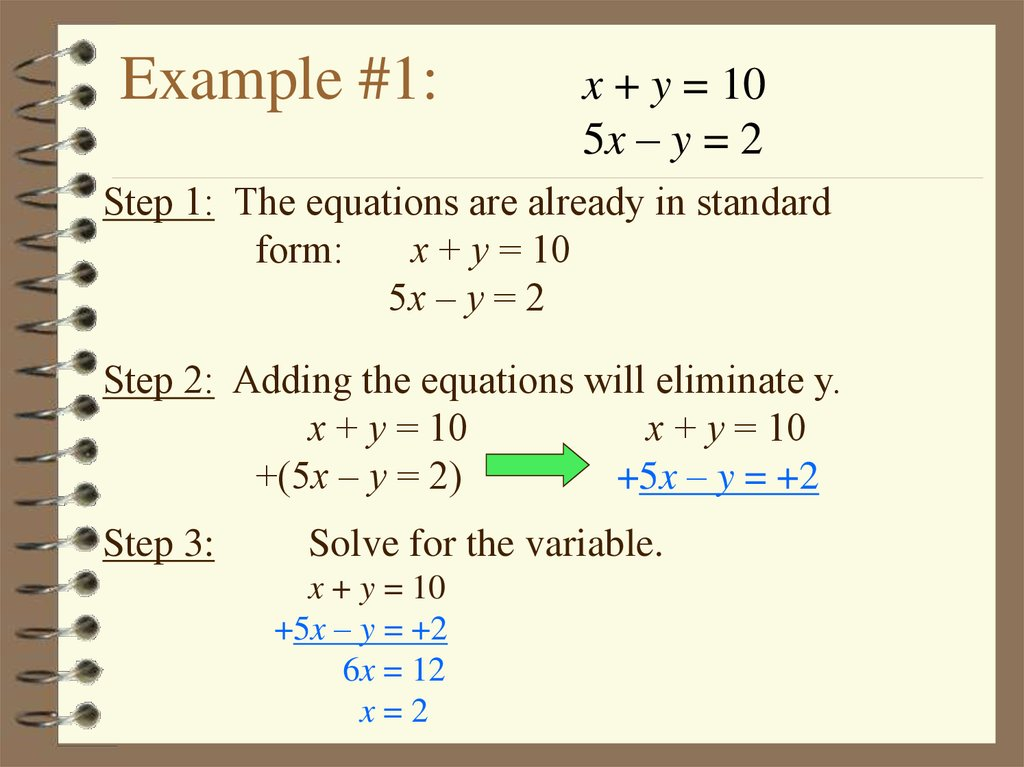# Solving Systems of Equations using Elimination

## 1. Solving Systems of Equations using Elimination

INF-21
Botakul Bakdaulet

## 2.

Steps:
1. Place both equations in Standard Form, Ax + By = C.
2. Determine which variable to eliminate with Addition
or Subtraction.
3. Solve for the remaining variable.
4. Go back and use the variable found in step 3 to find
the second variable.
5. Check the solution in both equations of the system.

## 3. Example #1:

x + y = 10
5x – y = 2
Step 1: The equations are already in standard
form:
x + y = 10
5x – y = 2
Step 2: Adding the equations will eliminate y.
x + y = 10
x + y = 10
+(5x – y = 2)
+5x – y = +2
Step 3:
Solve for the variable.
x + y = 10
+5x – y = +2
6x = 12
x=2

## 4.

x + y = 10
5x – y = 2
Step 4:
Solve for the other variable by
substituting into either equation.
x + y = 10
2 + y = 10
y=8
Solution to the system is (2,8).

## 5.

x + y = 10
5x – y = 2
Step 5:
Check the solution in both equations.
Solution to the system is (2,8).
x + y =10
2 + 8 =10
10=10
5x – y =2
5(2) - (8) =2
10 – 8 =2
2=2

1.
2.
2x + 4y =1
x - 4y =5
2x – y =6
x+y=3

## 7. Using Elimination to Solve a Word Problem:

Two angles are supplementary. The
measure of one angle is 10 degrees
more than three times the other.
Find the measure of each angle.

## 8. Using Elimination to Solve a Word Problem:

Two angles are supplementary. The
measure of one angle is 10 more
than three times the other. Find the
measure of each angle.
x = degree measure of angle #1
y = degree measure of angle #2
Therefore x + y = 180

## 9. Using Elimination to Solve a Word Problem:

Two angles are supplementary. The
measure of one angle is 10 more
than three times the other. Find the
measure of each angle.
x + y = 180
x =10 + 3y

Solve
x + y = 180
x =10 + 3y
x + y = 180
-(x - 3y = 10)
4y =170
y = 42.5
x + 42.5 = 180
x = 180 - 42.5
x = 137.5
(137.5, 42.5)

## 11. Now you Try to Solve These Problems Using Elimination.

Solve
1. Find two numbers whose sum is
18 and whose difference is 22.
2. The sum of two numbers is 128
and their difference is 114. Find
the numbers.